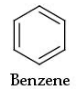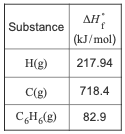# Problem: Part B. What is the chemical equation for the reaction that corresponds to breaking just the carbon-carbon bonds in gaseous benzene,C6H6(g)? Indicate the phase of each species in your answer.Express your answer as a chemical equation including phases.Determine the standard enthalpy change for the reaction that represents breaking all of the bonds in benzene, determine the equation for breaking all the carbon-carbon bonds of benzene use Hess's law to calculate the enthalpy change of this reaction and calculate the average bond enthalpy of a carbon-carbon bond in benzene This tutorial has been developed from Brown/LeMay/Bursten Integrative Exercise 8.105 and is a multiple-concept tutorial.Consider benzene in the gas phase, C6H6(g).Use the heat of formation, ΔH°f , values below to answer the questions.

###### FREE Expert Solution
83% (449 ratings)View Complete Written Solution
###### Problem Details

Part B. What is the chemical equation for the reaction that corresponds to breaking just the carbon-carbon bonds in gaseous benzene,C6H6(g)? Indicate the phase of each species in your answer.

Determine the standard enthalpy change for the reaction that represents breaking all of the bonds in benzene, determine the equation for breaking all the carbon-carbon bonds of benzene use Hess's law to calculate the enthalpy change of this reaction and calculate the average bond enthalpy of a carbon-carbon bond in benzene This tutorial has been developed from Brown/LeMay/Bursten Integrative Exercise 8.105 and is a multiple-concept tutorial.

Consider benzene in the gas phase, C6H6(g).Use the heat of formation, ΔH°f , values below to answer the questions.